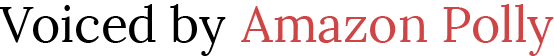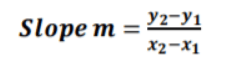# Teaching Slope-Intercept Form

 Would You Rather Listen to the Lesson?When learning about linear functions, algebra 1 students learn what the concept of slope-intercept form of a linear equation means, as well as how to represent any line using slope-intercept form.

Even though the slope-intercept form may not be the simplest lesson for students, there are many ways in which math teachers can make this topic more accessible and more engaging. Read on to find out how to teach slope-intercept form in a creative way.

## Tips for Teaching the Slope-Intercept Form

### What Are Linear Equations?

You can start your lesson on the slope-intercept form of a linear equation by first explaining what linear equations are. Define linear equations as equations that produce a straight line when we plot them on a graph.

Add that each term in a linear equation has an exponent of 1. Provide a few examples:

• y = 2x – 4
• 3x + 6y = 18
• 3x – 2y = 8
• y = 8x – 9

### What Is Slope-Intercept Form?

Point out to students that there are a lot of ways to represent a linear equation, among which the slope-intercept form is only one. So what is slope-intercept form? Explain that the slope-intercept form of a linear equation (or a line) is of the following form:

y = mx + b

…where m represents the slope of the line, and b is the y-intercept, that is, the y coordinate of the point where the line crosses the y-axis. Also remind students that the slope is what indicates the line steepness, so with a greater slope, the steepness is also greater.

#### Example:

Write an equation on the whiteboard and demonstrate to students how to find the slope and y-intercept. For instance, you can write the following equation:

y = – 4x – 5

Then, tell students to compare the above equation with the mentioned form:

y = mx + b

If we draw a comparison between the two, point out that we can observe that m equals – 4 and b equals – 5. In other words, the slope is – 4 and the y-intercept is – 5.

#### Launch a Video!

Use this video by Khan Academy to illustrate how to graph a linear equation. The video shows how to graph y = 2x + 3 in the coordinate plane, and then find the y-intercept as well as the slope.

Add that the y-intercept is 3 and the slope is 2 in y = 2x + 3 from what we can observe in the graph as well. By creating a table with values for x and y, we can see that when x is 0, y is 3; when x is 1, y is 5; when x is 2, y is 7.

Explain that our change in y is 2, that is, for every 1 that x increased, y increased by 2. Add that in the linear equation at hand, y = 2x + 3, the change in y equals 2 when the change in x equals 1. We can also present in the following way:### Slope-Intercept Form Given Two Points

Explain to students that when we are given two points on a line, we can write their slope-intercept form by following these steps:

• First, we will find the slope with the help of the following slope formula:• Then, we’ll find the y-intercept by replacing the values of any of the two points (x1, y1) or (x2, y2) in the formula y = mx + b and then we’ll solve for b.
• Finally, we’ll write the final equation by using the values for m and b that we already found in the above steps.

#### Example:

Present an example of how to write an equation in slope-intercept form when we’re given two points. For instance, let’s say we’re given the following points (1, – 3) and (3, 1) and we need to write an equation in slope-intercept form for the line going through these points.

By following the above-mentioned steps, first, we’ll find the slope m with the slope formula. By replacing the values for (x1, y1) and (x2, y2) and we’ll get:So slope m is 2. Now that we’ve determined it, we’ll put the slope m together with one of the two points in y = mx + b and solve for b. Let’s say we’ll put the point (3, 1) in y = mx + b, which will result in the following:

1 = 2(3) + b

1 = 6 + b

1 – 6 = b

b = – 5

Finally, we’ll write the equation using the values for m and b that we’ve already identified:

y = mx + b

y = 2x – 5

## Activities to Practice Slope-Intercept Form

### Graph from Slope-Intercept Form

This exercise will help students reinforce their skills to graph a linear equation in slope-intercept form. To implement this activity in your class, make sure all students have a suitable device (one per student).

Students do this exercise individually. In each question, they’re presented with an equation in slope-intercept form and asked to graph it by first finding the slope and then using this slope to find a second point.

If they get stuck, students can also choose to use a hint or watch a video as help for each question. In the end, open space for discussion and reflection. How did students identify the slope and the first point? How did they identify the second point for the graph?

### Group Work

This activity will help students practice slope-intercept form via diverse exercises, as well as reinforce peer-to-peer tutoring. To implement this activity in your class, you’ll need to print out this Assignment Worksheet (Members Only).

Divide students into pairs and explain that each pair works together. The worksheet contains exercises where students have to find the slope and y-intercept of an equation, write an equation in slope-intercept form of the line passing through two given points, etc.

In the end, ask the pairs to present their work. Which exercises were the most challenging? Which steps did they follow to write an equation in slope-intercept form of the line that passes through the given points?

Before You Leave…

If you liked the tips on how to teach slope-intercept form in a creative way that we outlined in this article, we have a whole lesson that is dedicated to this topic!

So if you’re looking for more math materials for children of all ages, sign up for our emails for plenty of free content! Feel free to also head over to our blog, and check out our free resources on teaching functions!

And if you’d like to become a member, sign up at Math Teacher Coach!